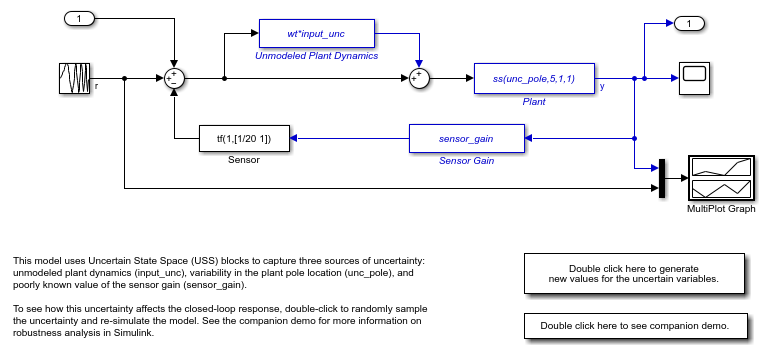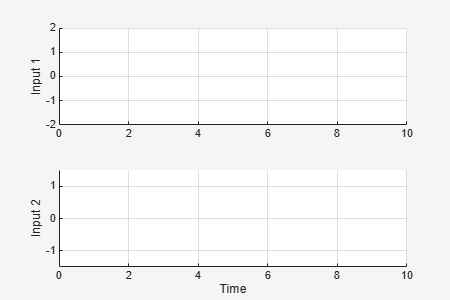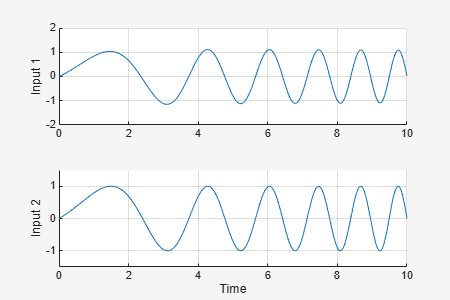# usample

Generate random samples of uncertain variables in a Simulink model

## Syntax

```samples = usample(uvars,N)
samples = usample(uvars)
samples = usample(uvars,N,Wmax)
```

## Description

This function is for generating random samples of uncertain variables stored in a data structure you obtain from a Simulink® model, using `ufind`. To generate random samples from uncertain models (`uss`, `ufrd`) or generalized state-space models (`genss`, `genfrd`), use `usample (uss)`.

`samples = usample(uvars,N)` generates `N` random samples of the uncertain variables in `uvars`. `uvars` is a structure that lists uncertain variables (`ureal`, `ucomplex`, `umargin`, or `ultidyn`) by name. You can automatically obtain `uvars` for a Simulink model that contains Uncertain State Space blocks using `ufind`. `samples` is an N-by-1 structure array whose field names and values are the names and sample values of the uncertain variables. Use this syntax, together with `ufind`, to generate random samples for uncertain variables in Simulink models.

`samples = usample(uvars)` is equivalent to `usample(uvars,1)`.

`samples = usample(uvars,N,Wmax)` specifies constraints, as described in `uss/usample`, for sampling uncertain variables of type `ultidyn` in `uvars`.

## Examples

collapse all

Create a structure that contains uncertain variables `a` and `b`.

`uvars = struct('a',ureal('a',5),'b',ultidyn('b',[2 3],'Bound',7))`
```uvars = struct with fields: a: [1x1 ureal] b: [2x3 ultidyn] ```

Generate a random sample of `a` and `b`.

`samples = usample(uvars)`
```samples = struct with fields: a: 5.6294 b: [2x3 ss] ```

`samples` is also a structure, where each field contains a randomly sampled instance of the uncertain variable type in `uvars`. You can use `samples` to simulate an uncertain Simulink model at these values as shown in Sample Uncertain Variables in a Simulink® Model.

Generate random samples of uncertain variables in a Simulink® model.

Open the model.

```open_system('usim_model') ```The model contains three Uncertain State Space blocks named Unmodeled Plant Dynamics, Plant, and Sensor Gain. These blocks depend on three uncertain variables named `input_unc`, `unc_pole`, and `sensor_gain`.

Use `ufind` to find all Uncertain State Space blocks and uncertain variables in the model.

```uvars = ufind('usim_model'); ```Use `usample` to generate random samples of `input_unc`, `unc_pole`, and `sensor_gain`. Simulate the closed-loop response for each of these random samples.

```for i=1:10; uval = usample(uvars); sim('usim_model',10); end ```The MultiPlot Graph block displays the simulated responses.

## How To